Inductor

Inductor is an electrical component that stores energy in magnetic field.

The inductor is made of a coil of conducting wire.

In an electrical circuit schematics, the inductor marked with the letter L.

The inductance is measured in units of Henry [L].

Inductor reduce current in AC circuits and short circuit in DC circuits.

Inductor picture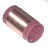Inductor symbols

Inductor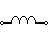Iron core inductor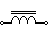Variable inductor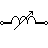Inductors in series

For several inductors in series the total equivalent inductance is:

LTotal = L1+L2+L3+...

Inductors in parallel

For several inductors in parallel the total equivalent inductance is: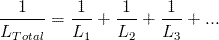Inductor's voltage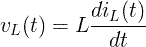Inductor's current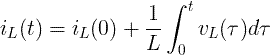Energy of inductor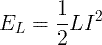AC circuits

XL = ωL

Inductor's impedance

Cartesian form:

ZL = jXL = jωL

Polar form:

ZL = XL∠90º

Currently, we have around 1977 calculators, conversion tables and usefull online tools and software features for students, teaching and teachers, designers and simply for everyone.